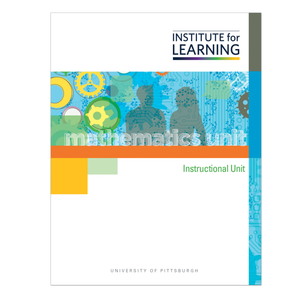# Grade 4: Multiply and Divide with Flexible Strategies (CCSSM Version)

Regular price \$149.00 \$0.00 Unit price per

Concepts

• Basic Multiplication of Two-, Three-, and Four-Digit Factors by One-Digit Factor
• Basic Division of Two-, Three-, and Four-Digit Dividends by One-Digit Divisor
• Multiplication of a Two-Digit Factor by a Two-Digit Factor

Unit includes

• 24 high-level tasks with associated QuickStarts, Practices, Applications, and Quick Writes.
• 3 checkpoints and 6 support activities.
• 1 final performance-based assessment with scoring guide.

Students

• Develop and use flexible strategies for solving multiplication situations, including
• Applying the distributive property of multiplication over addition,
• Using rounding and compensating, and
• Factoring a factor as a means for solving situations involving two-digit factors.
• Solve multiplication equations and situations involving up to a four-digit factor multiplied by a one-digit factor.
• Solve equations and situations involving a two-digit factor multiplied by a two-digit factor.
• Develop and use flexible strategies for solving division equations and situations involving up to a four-digit factor dividend divided by a one-digit divisor, including:
• Using the distributive property of division over addition and
• Using the relationship between multiplication and division.
• Use known facts to multiply and divide larger quantities.
• Use place value to strategically decompose quantities to make multiplying and dividing easier.